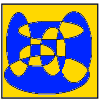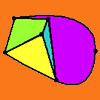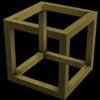# Search by Topic

Filter by: Content type:
Age range:
Challenge level:

### There are 4 results

Broad Topics > Decision Mathematics and Combinatorics > Euler's formula### Painting by Numbers

##### Age 16 to 18 Challenge Level:

How many different colours of paint would be needed to paint these pictures by numbers?### Euler's Formula

##### Age 16 to 18

Some simple ideas about graph theory with a discussion of a proof of Euler's formula relating the numbers of vertces, edges and faces of a graph.### Impossible Polyhedra

##### Age 16 to 18

Is it possible to make an irregular polyhedron using only polygons of, say, six, seven and eight sides? The answer (rather surprisingly) is 'no', but how do we prove a statement like this?### Euler's Formula and Topology

##### Age 16 to 18

Here is a proof of Euler's formula in the plane and on a sphere together with projects to explore cases of the formula for a polygon with holes, for the torus and other solids with holes and the. . . .